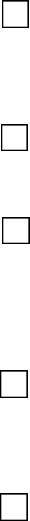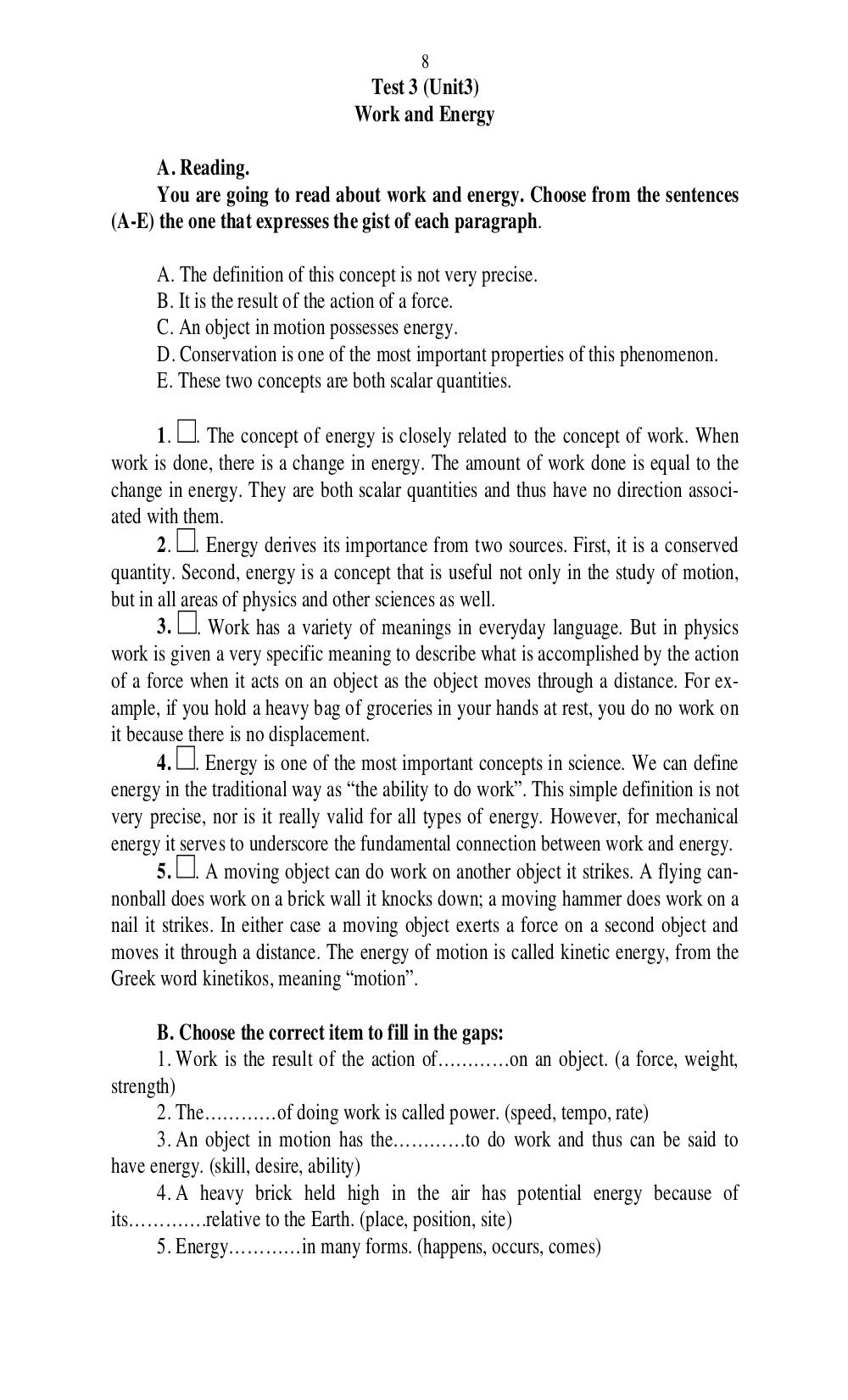# Английский язык. Ч. 1. Ильичева Н.А - 8 стр.

Составители:

Рубрика:

• ## Иностранный язык8
Test 3 (Unit3)
Work and Energy
You are going to read about work and energy. Choose from the sentences
(A-E) the one that expresses the gist of each paragraph.
A. The definition of this concept is not very precise.
B. It is the result of the action of a force.
C. An object in motion possesses energy.
D. Conservation is one of the most important properties of this phenomenon.
E. These two concepts are both scalar quantities.
1. . The concept of energy is closely related to the concept of work. When
work is done, there is a change in energy. The amount of work done is equal to the
change in energy. They are both scalar quantities and thus have no direction associ-
ated with them.
2. . Energy derives its importance from two sources. First, it is a conserved
quantity. Second, energy is a concept that is useful not only in the study of motion,
but in all areas of physics and other sciences as well.
3. . Work has a variety of meanings in everyday language. But in physics
work is given a very specific meaning to describe what is accomplished by the action
of a force when it acts on an object as the object moves through a distance. For ex-
ample, if you hold a heavy bag of groceries in your hands at rest, you do no work on
it because there is no displacement.
4. . Energy is one of the most important concepts in science. We can define
energy in the traditional way as the ability to do work. This simple definition is not
very precise, nor is it really valid for all types of energy. However, for mechanical
energy it serves to underscore the fundamental connection between work and energy.
5. . A moving object can do work on another object it strikes. A flying can-
nonball does work on a brick wall it knocks down; a moving hammer does work on a
nail it strikes. In either case a moving object exerts a force on a second object and
moves it through a distance. The energy of motion is called kinetic energy, from the
Greek word kinetikos, meaning motion.
B. Choose the correct item to fill in the gaps:
1. Work is the result of the action of……on an object. (a force, weight,
strength)
2. The…………of doing work is called power. (speed, tempo, rate)
3. An object in motion has the……to do work and thus can be said to
have energy. (skill, desire, ability)
4. A heavy brick held high in the air has potential energy because of
its……….relative to the Earth. (place, position, site)
5. Energy…………in many forms. (happens, occurs, comes)8
Test 3 (Unit3)
Work and Energy

You are going to read about work and energy. Choose from the sentences
(A-E) the one that expresses the gist of each paragraph.

A. The definition of this concept is not very precise.
B. It is the result of the action of a force.
C. An object in motion possesses energy.
D. Conservation is one of the most important properties of this phenomenon.
E. These two concepts are both scalar quantities.

1. . The concept of energy is closely related to the concept of work. When
work is done, there is a change in energy. The amount of work done is equal to the
change in energy. They are both scalar quantities and thus have no direction associ-
ated with them.
2. . Energy derives its importance from two sources. First, it is a conserved
quantity. Second, energy is a concept that is useful not only in the study of motion,
but in all areas of physics and other sciences as well.
3. . Work has a variety of meanings in everyday language. But in physics
work is given a very specific meaning to describe what is accomplished by the action
of a force when it acts on an object as the object moves through a distance. For ex-
ample, if you hold a heavy bag of groceries in your hands at rest, you do no work on
it because there is no displacement.
4. . Energy is one of the most important concepts in science. We can define
energy in the traditional way as “the ability to do work”. This simple definition is not
very precise, nor is it really valid for all types of energy. However, for mechanical
energy it serves to underscore the fundamental connection between work and energy.
5. . A moving object can do work on another object it strikes. A flying can-
nonball does work on a brick wall it knocks down; a moving hammer does work on a
nail it strikes. In either case a moving object exerts a force on a second object and
moves it through a distance. The energy of motion is called kinetic energy, from the
Greek word kinetikos, meaning “motion”.

B. Choose the correct item to fill in the gaps:
1. Work is the result of the action of…………on an object. (a force, weight,
strength)
2. The…………of doing work is called power. (speed, tempo, rate)
3. An object in motion has the…………to do work and thus can be said to
have energy. (skill, desire, ability)
4. A heavy brick held high in the air has potential energy because of
its………….relative to the Earth. (place, position, site)
5. Energy…………in many forms. (happens, occurs, comes)